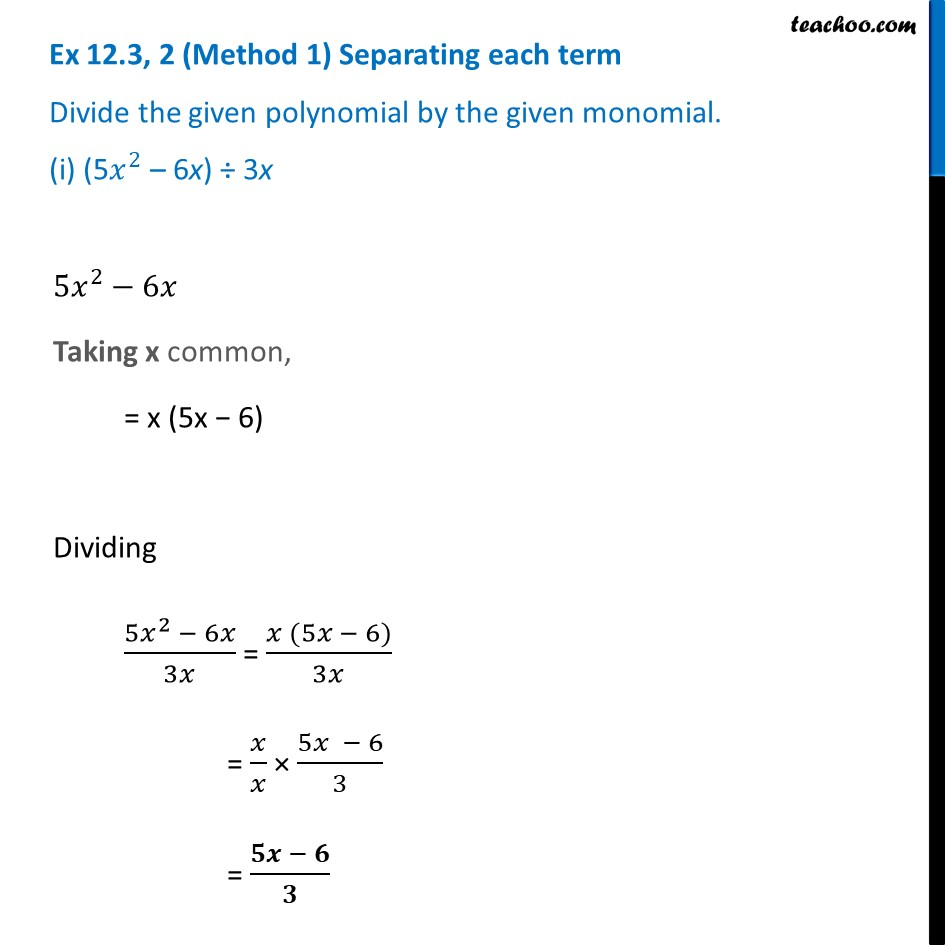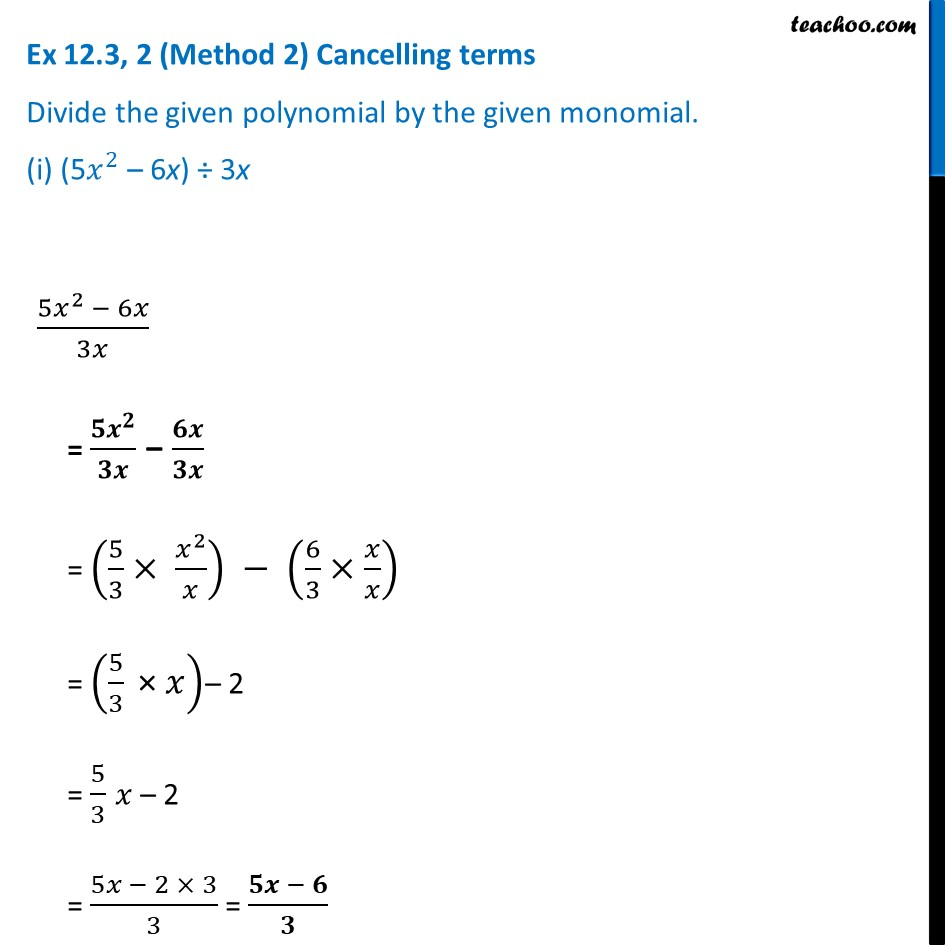Ex 12.3

Chapter 12 Class 8 Factorisation
Serial order wiseLearn in your speed, with individual attention - Teachoo Maths 1-on-1 Class

### Transcript

Ex 12.3, 2 (Method 1) Separating each term Divide the given polynomial by the given monomial. (i) (5𝑥^2 – 6x) ÷ 3x 〖5𝑥〗^2−6𝑥 Taking x common, = x (5x − 6) Dividing (5𝑥^2 − 6𝑥)/3𝑥 = (𝑥 (5𝑥 − 6))/3𝑥 = 𝑥/𝑥 × (5𝑥 − 6)/3 = (𝟓𝒙 − 𝟔)/𝟑 Ex 12.3, 2 (Method 2) Cancelling terms Divide the given polynomial by the given monomial. (i) (5𝑥^2 – 6x) ÷ 3x(5𝑥^2 − 6𝑥)/3𝑥 = (𝟓𝒙^𝟐)/𝟑𝒙 − 𝟔𝒙/𝟑𝒙 = (5/3× 𝑥^2/𝑥) − (6/3×𝑥/𝑥) = (5/3 " × " 𝑥)– 2 = 5/3 𝑥 – 2 = (5𝑥 − 2 × 3)/3 = (𝟓𝒙 − 𝟔)/𝟑